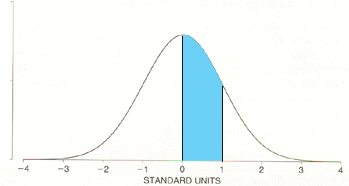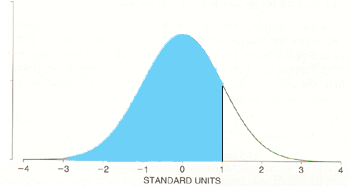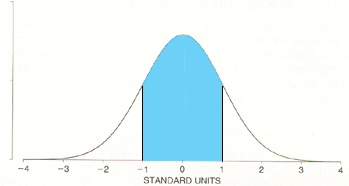SEARCH HOMEMath Central Quandaries & QueriesQuestion from GEORGE, a teacher: Statistics texts state that in a normal distribution, 1 standard deviation covers 68%, 2SD 95% 3SD 99.7%. However, on looking at the tables for normal curve areas, the percentage for a z value of 1 is 84.13. 68 % of values are covered at a z value of 0.47. The discrepancies exist even for other values but are smaller. So why is the rule of thumb so different from the Table for Normal Curve areas?Hi George,

When I look up a z value of 1 in my table of standard normal table I get the value of Pr(0 < z < 1) as in the diagram below, and the value in the table is 0.3413, or if you prefer 34.13%.Pr(0 < z < 1)

It looks like you have added 50% to this value to obtain 84.13%. That would give you Pr( z < 1).Pr( z < 1)

When the statistics texts state that "in a normal distribution, 1 standard deviation covers 68%" they mean that 68% of the probability (area) is between -1 and 1.Pr(-1 < z < 1)

To find the value of Pr(-1 < z < 1) from the table you need to double the value of Pr(0 < z < 1). Hence the value is 2 × 0.3413 = 0.6826 or approximately 68%.

I hope this helps,
HarleyMath Central is supported by the University of Regina and The Pacific Institute for the Mathematical Sciences.